• 검색 결과가 없습니다.

# Seong Bo Kang

N/A
N/A
Protected

Share "Seong Bo Kang"

Copied!
52
0
0
더 보기 ( 페이지)

전체 글

Background

## Theoretical & mathematical development

In this work, we present the basic elastic equation and present the solution with scalar and vector potential. It will be shown that two basic types of waves, longitudinal waves and shear waves, can propagate in infinite media characterized by definite velocities. -4) talks about the equation of motion in this equation b is body force and 𝜌 is density.

In equation (2-5), 𝜆 and 𝜇 Lame parameters, Lame modulus and shear modulus, respectively, are defined as E and 𝜈. Therefore, to express the equation uniquely, the calibration condition is used to express it uniquely. In this equation (2-11), some parts of the equation become zero due to vector identities and gauge condition.

In equation (2-12), the two terms 𝜙, 𝜓 are independent, so each term becomes zero as shown below.

• Diatomic chain system
• Mass in mass system

A mass-in-mass system with local resonator located inside arranged is shown in Fig 2-4. Equation of motion of the periodic mass in mass system is written as Eq. Assume the harmonic wave and flake-bloch condition, . -28) The distribution curve of diatomic system and mass-in-mass system is shown in Fig 1-2.

### Three points method

In the presence of metamaterials, the transmission becomes close to zero in the stopping frequency band of the bandgap, as shown in Figure 2-6(b). The position 𝑥𝑖 of the ith particle is updated by adding the velocity 𝑣𝑖 of the particle, as shown in equation (3.1). We update the position 𝑥𝑖 of the particle using the velocity 𝑣𝑖(𝑚+1) calculated in the previous step.

On the other hand, the mode shape of the shear wave is not symmetric on the axis. As in the previous optimization, the design variables were set as the outer diameter R and the inner diameter r of the cylinder. As shown in Fig. 3-10, with the center frequency of the bandgap as the objective function, the objective function is the same as Eq (3-7), and a flowchart of the optimization is shown in Fig. 3-8 (b). .

According to Figure 3-11, the center frequency of the band gap obtained by optimization is 5000. According to Figure 3-14, the center frequency of the band gap obtained by optimization is close to 3000 Hz. First, in the case of width size optimization, the simulation results are as follows.

Second, in the case of the size optimization position at the bandpass frequency (4500 Hz, 5000 Hz) other than the band gap region, the wave is propagated by the metamaterial. In the case of a shear wave, the simulation setup is as follows in Figure 4-4. Second, in the case of the size optimization position at the bandpass frequency (1500 Hz, 2000 Hz) other than the band gap region, the wave is propagated by the metamaterial.

We compared the amplitude of the wave propagating the structure without the metamaterial (P1) and the case of propagating the metamaterial (P2). In the size optimization design, the width of the band gap was optimized using the PSO algorithm, and the optimization was performed to position the band gap at the desired position. The band gap of the metamaterial obtained by PSO was validated by finite element analysis in COMSOL Multiphysics.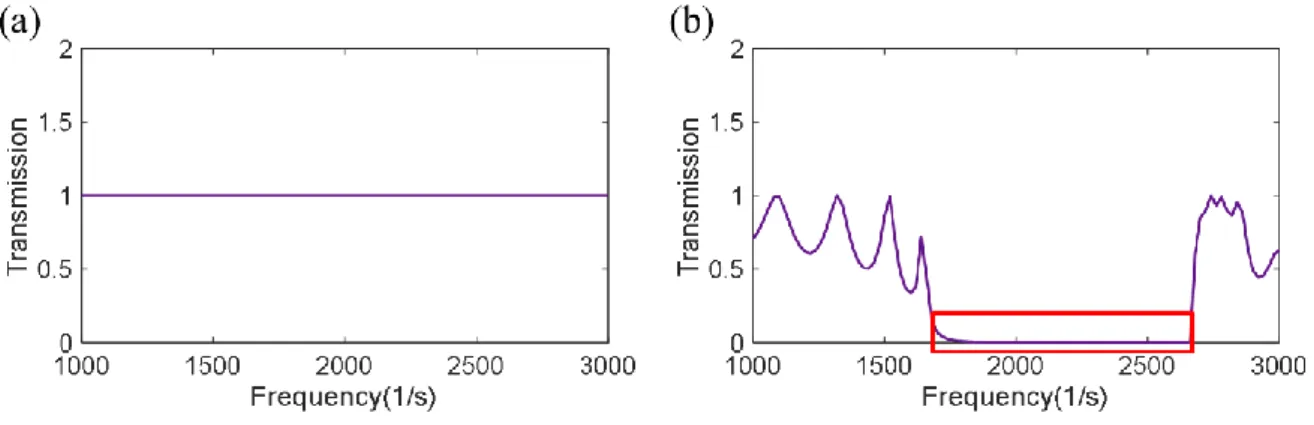Fig 2-6 (a) full transmission at same medium (b) transmission of metamaterial bandgap

## Numerical Simulation & Optimization

### Particle Swarm Optimization (PSO)

Therefore, the structural design is carried out for the purpose of size optimization by adjusting the outer diameter and inner diameter by performing finite element analysis on the shape using sufficient grids as a simple structure. Swarm-based optimization is a method of mathematical optimization in which several optimizers exchange information with each other while performing optimization. The Particle Swarm Optimization (PSO) algorithm, inspired by the social behavior model of bioswarm such as bees or birds, ants and fish, is one of the representative optimization algorithms for complex optimization problems .

After initializing the algorithm, three processes are repeated calculating the velocity, updating the position, and evaluating the solution to find the optimal solution. Next, we calculate the velocity next time for the particle's current position and velocity. In Equation (3-2) 𝑝𝑖 is the best solution obtained by the ith particle searching the solution space up to the current time k-1, G is the best solution found in the entire swarm to date.

With this update method, even if a particle falls into the local optimum in PSO, it can escape from the local minimum using g, which is the information of all particles. As in equation 3.3, the solution is updated while the newly calculated velocity is added to the current position. If the goal is to minimize the objective function, update 𝑝i to 𝑥𝑖(𝑚+1) if Eq (3.4) is satisfied and test Eq (3.5) for the updated 𝑝i.

At the end of the evaluation phase, review the established termination conditions and complete the PSO if the termination conditions are met. If not, return to the speed calculation and repeat the steps of speed calculation, position update and evaluation. However, in this article, the band gap is not increased arbitrarily, but the target frequency is set to obtain the band gap width in the desired band. Later, research will be conducted by expanding to multi-objective optimization.

### Dispersion curve

So an analytical technique to obtain a dispersion curve corresponding to longitudinal waves and shear waves was found. By analyzing the dispersion curve in fig. 3-3, we found that the branch corresponding to the longitudinal wave and shear wave in the complete model of the dispersion curve was almost perfectly matched compared to the dispersion curve of the half model. If the shape of the unit cell changes, there is a limitation that it is difficult to use this method.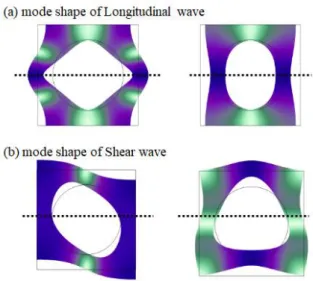Fig 3-2 (a) mode shape of Longitudinal wave (b) mode shape of Shear wave

### Longitudinal wave dispersion curve

• Longitudinal wave bandgap Optimization

The design variables are set as outer diameter R and inner diameter r and the width of the band gap to be achieved is set to 1500, and size optimization is performed. Optimization was performed in Matlab and finite element analysis was performed in COMSOL Multiphysics, and the results were exchanged and updated. As shown in Fig 3-7, with the bandgap width as the objective function, the objective function is the same as Eq (3-6) and a flowchart for the optimization is shown in Fig 3-8.

The results obtained through size optimization from PSO are outer diameter R = 25.86 mm and inner diameter r = 13.75 mm. It can be seen that the optimization is well done with a value that fits the target of 1500. Second, to make a band gap near the desired frequency band, the center frequency of the band gap was moved, and the band gap was adjusted to be located near the desired frequency band. desired frequency.

The results obtained through size optimization from PSO are outer diameter R = 27.41 mm and inner diameter r = 19.1 mm. It can be seen that the optimization is well done with a value that fits the target of 5000hz.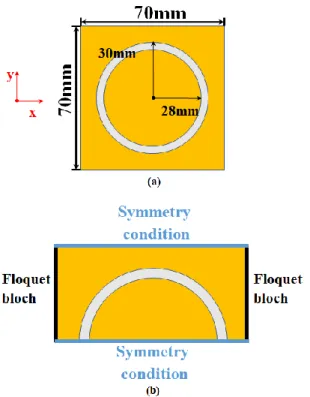Fig 3-5 (a)Numerical simulation model of a unit cell (b) simulation setting of longitudinal wave

### Shear wave dispersion curve

• Shear wave bandgap Optimization

The central frequency of the bandgap is set to 3000 Hz, and the objective function is set to find the optimal solution accordingly. After designing the metamaterial of outer diameter R and inner diameter r obtained through optimization, finite element analysis was used to verify if waves were well blocked at frequencies in the stopband of the bandgap. The simulation setup is shown in Fig 4-1. To prevent the wave from being reflected infinitely, we have given PML conditions on both sides of the model.

On the other hand, at the stopband frequency (4500hz, 5000hz) within the bandgap, the wave does not transmit the metamaterial, and the mode shape is shown in Fig 4-2. Moreover, it can be seen that the wave is well shielded because the transmission is very low at the bandgap frequency. On the other hand, at the stopband frequency (5000hz, 5500hz) within the bandgap, the wave does not transmit the metamaterial, and the mode shape, Transmission is shown in Fig 4-3.

On the other hand, at the stopband frequency (4000 Hz, 4500 Hz), inside the band gap, the wave does not transmit the metamaterial, and the mode shape is shown in Figure 4-5. On the other hand, at the stopband frequency (2500 Hz, 3000 Hz), inside the band gap, the wave does not transmit the metamaterial, and the Transmission mode shape is shown in Figure 4-6. In the case of a shear wave, if there is no metamaterial, the amplitude is 1, and when it passes through the metamaterial, the amplitude value is very low.

Therefore, it can be seen that the wave cannot be transmitted at the stopband frequency in the band gap based on the optimization. In this paper, we present a technique for the design of longitudinal wave band and shear wave band based on optimization. Through optimization, we can adapt the scale, expected to protect waves or reduce vibrations in large mechanical systems, such as vehicles, aircraft as well as in seismic applications.

And if we design from a 70mm scale to a larger scale, we can lower the frequency band. In the future, it is expected that metamaterials can be applied not only to longitudinal wave and shear wave, but also to other types of designed waves.

## Simulation validation

Longitudinal wave simulation validation

Shear wave simulation validation

Transient simulation

Conclusion

Acknowledgement

수치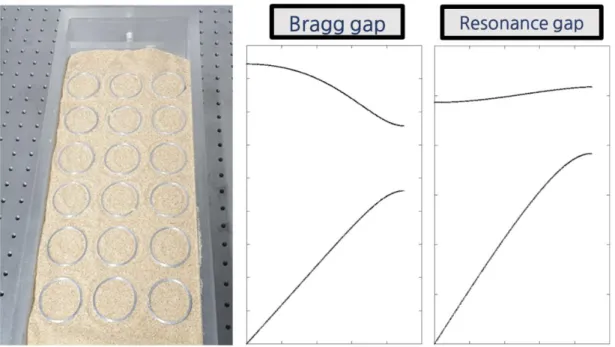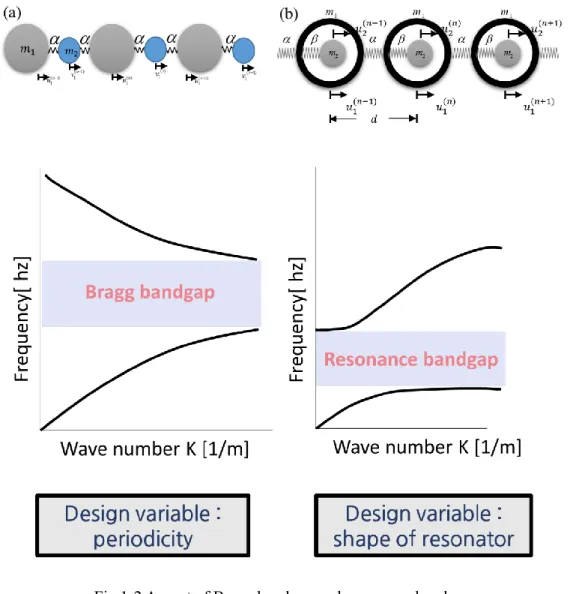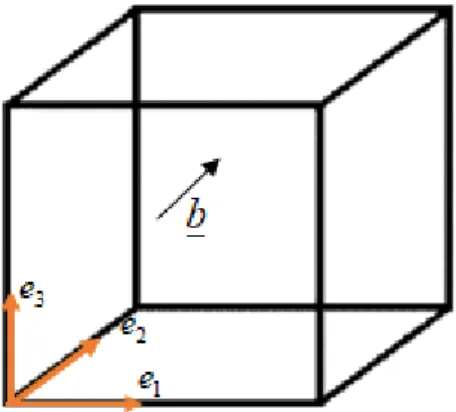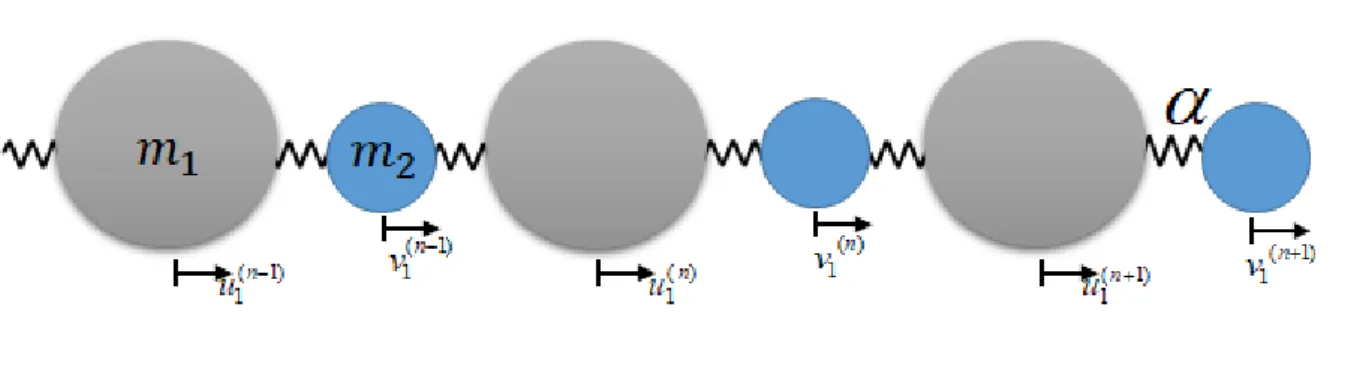+7

참조

관련 문서

This paper presents the Internet-based monitoring and control architecture and development of LonWorks control modules for AHU (air handling units) of HVAC in viewpoint

However, the problem in fixed hysteresis band delta modulation technique is a variation of switching frequency over the fundamental period of the modulation signal.. This

본 연구는 water, water+methyl alcohol solution, water+ethyl alcohol solution, water+1-prophyl alcohol solution, water+2-prophyl alcohol solution에서의 calcium

The constructed six-axis motion platform can perform lateral and vertical displacements and roll, pitch, and yaw motions similar to the 6-DOF motions of a tilting train while

We examined the level fluctuation of scintillation that occurred in the observation period from January, 2002 to December, 2002 in term of peak to peak values and S 4 index

Therefore , frequency control through the microcontroller is simple and practical frequency band are wider when comparing to application of 8-bit PWM

Erecta fig is an indigenous plant which belongs to the fig tree genus of Moraceae, and it is distributed in the southern coast and Jeju-do of Korea, while being called

Simulation waveforms at startup of the suggested converter employing 1-SL cells and 2-stage

à the equilibrium constant for reaction with HCl is.. § Strong base reacts “completely” with a weak acid. à because the equilibrium constant is, again, very large. § If HA

언어 선택하기

웹사이트는 선택한 언어로 번역됩니다.

기타 언어